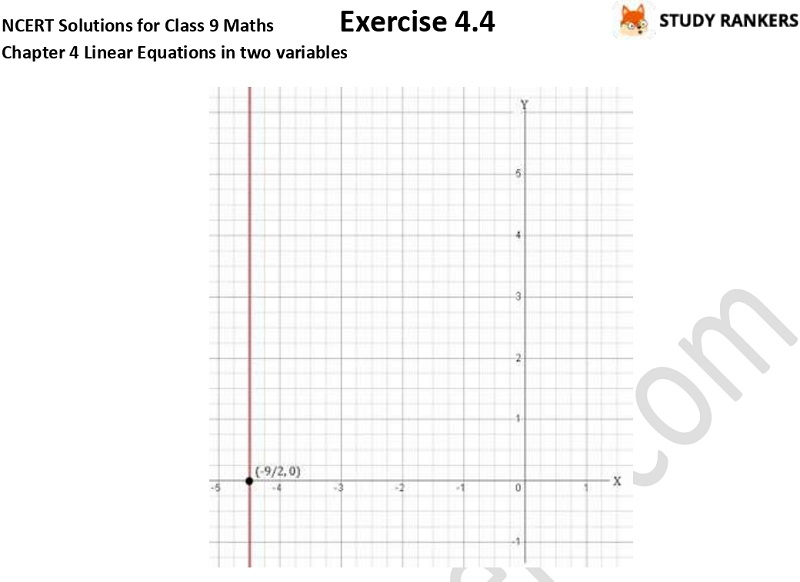>

## NCERT Solutions for Class 9 Maths Chapter 4 Linear Equations in Two Variables Exercise 4.4

Chapter 4 Linear Equations in Two Variables Exercise 4.4 Class 9 Maths NCERT Solutions will help you in completing your homework on time. NCERT Solutions for Class 9 Maths will prove very useful in completing home assignments on time. These NCERT Solutions are updated as per the latest marking scheme released by CBSE. You can revise the concepts through these questions and answers and excel in your exams.

Exercise 4.4 has only two questions in which you have to give the geometric representations of y = 3 as an equation.